# 识别器

## 识别单词

c <- NextChar()
if c = 'n'
c <- NextChar()
if c = 'e'
c <- NextChar()
if c = 'w'
return 'success'
else
throw 'error'
end
else
throw 'error'
end
else
throw 'error'
end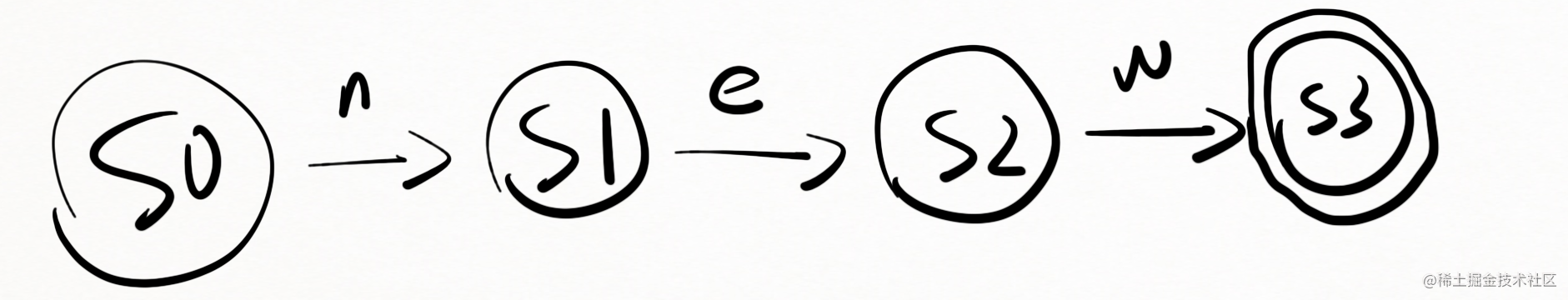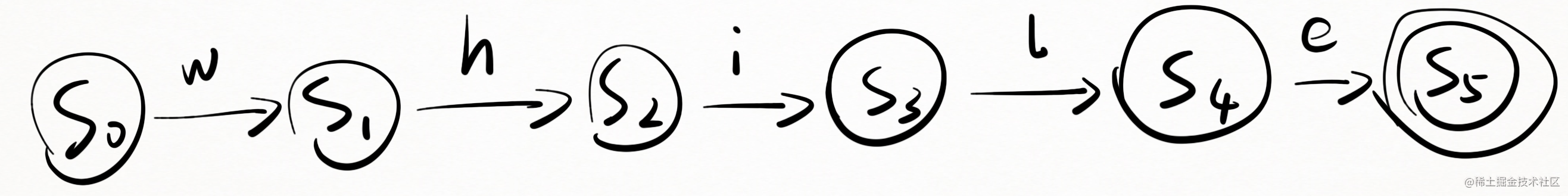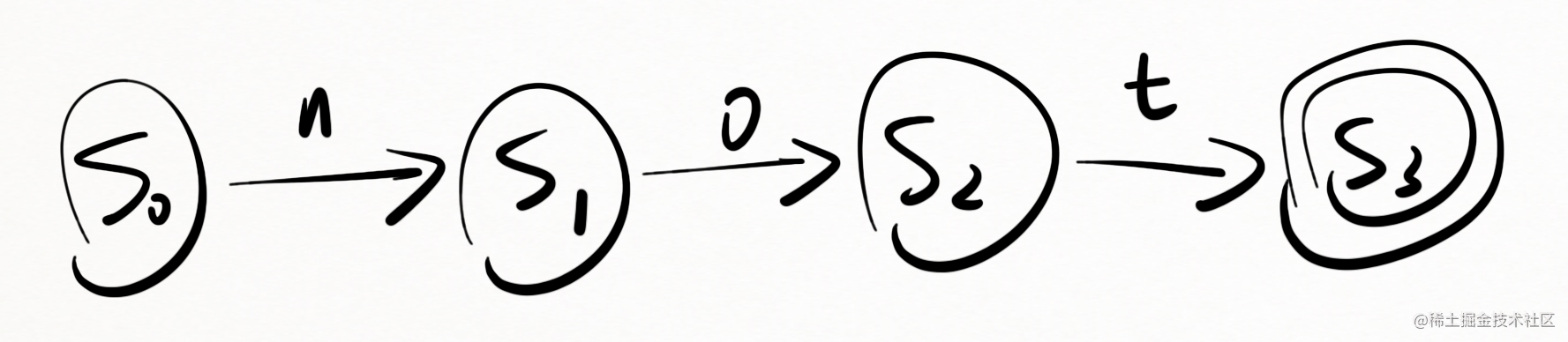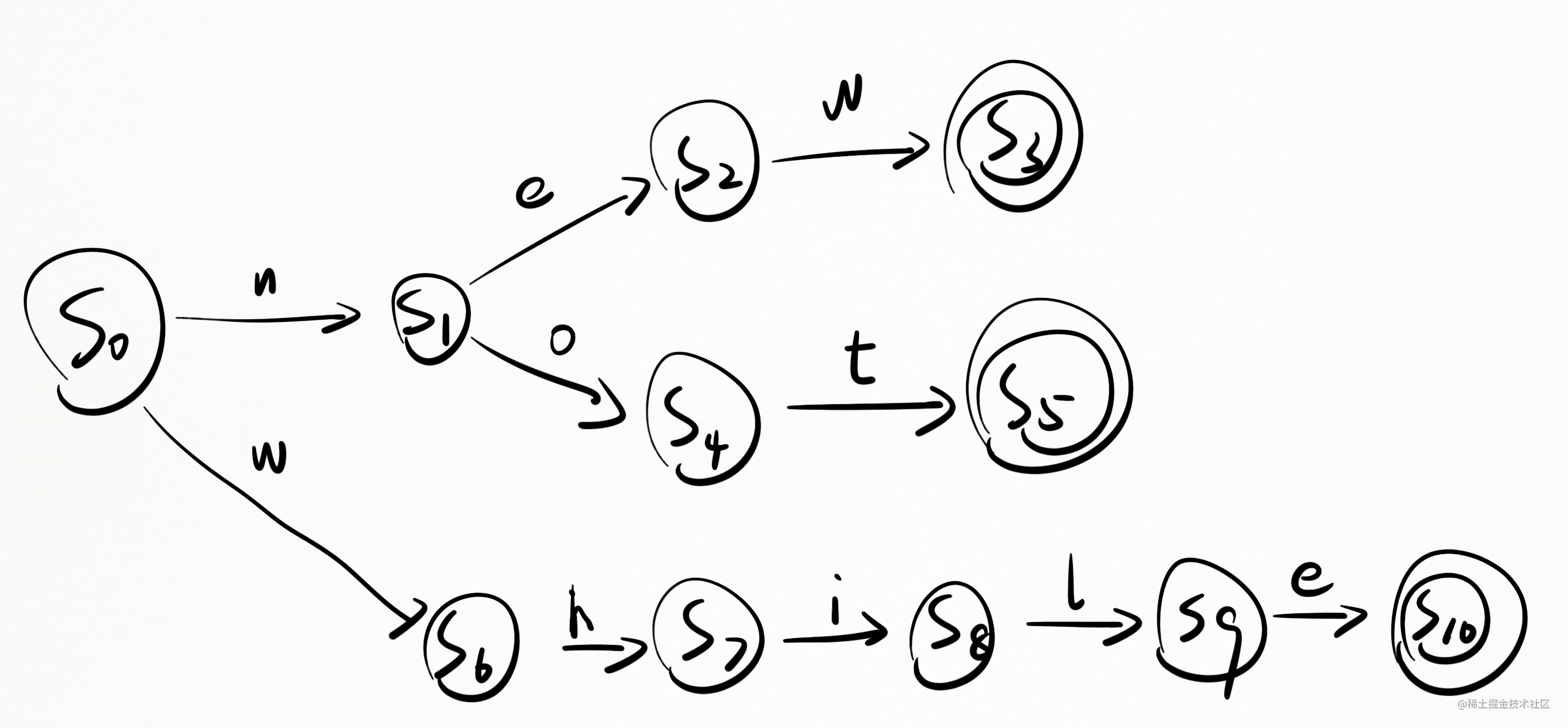## 识别器的形式化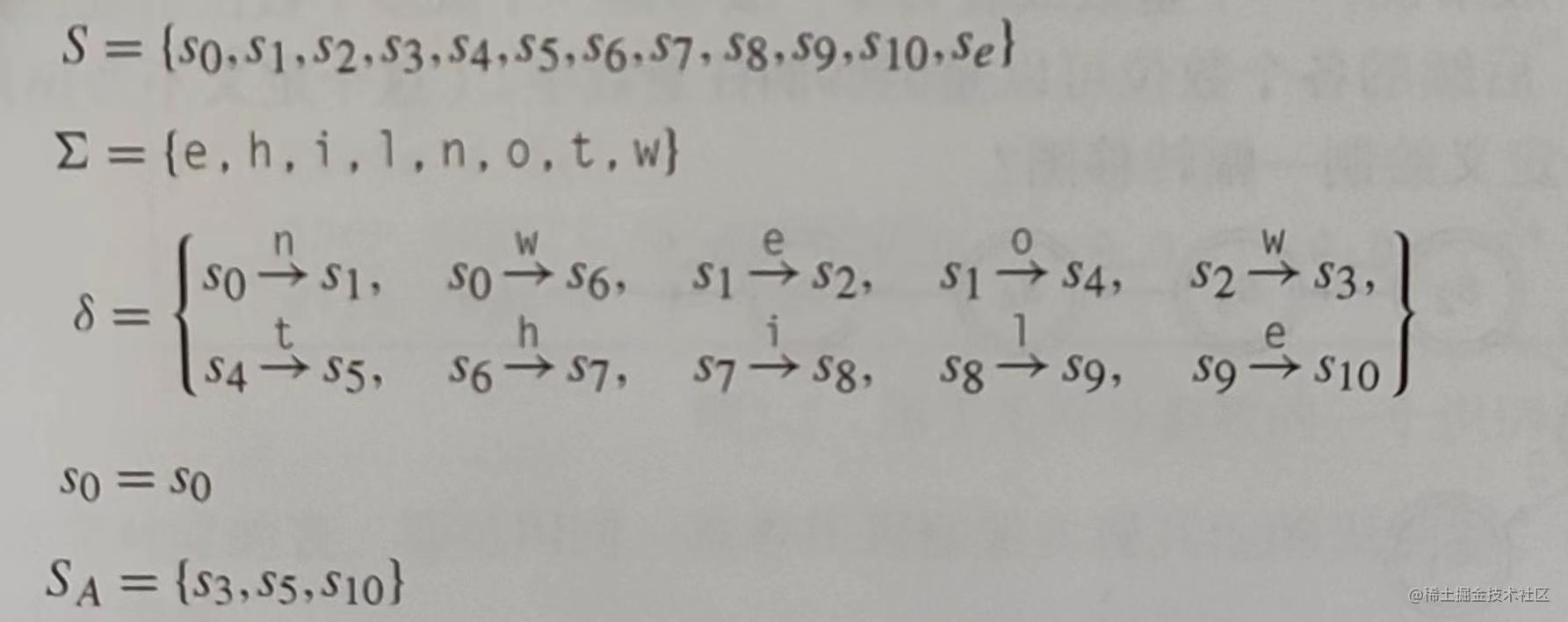• $S$ 表示所有状态，其中 $s_e$ 是错误状态，转移图一般不画 $s_e$
• $\Sigma$ 是识别器使用的字母表
• $\delta$ 是识别器的所有转移函数
• $s_0$ 是初始状态
• $S_A$ 是接受状态的集合

## 识别数字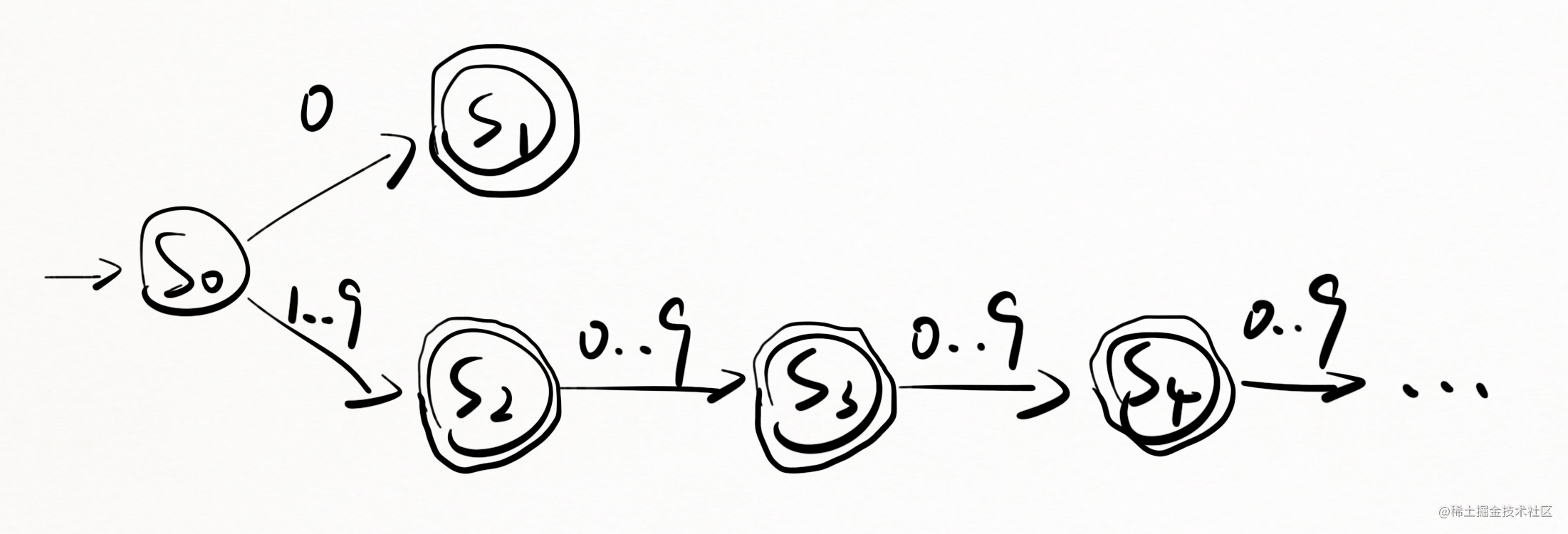1. 它无法终结，这违反了 $S$ 是有限集的定义。
2. $S_2$ 开始，所有的状态都是等价的，因为他们期待同样的数字切均为接受状态。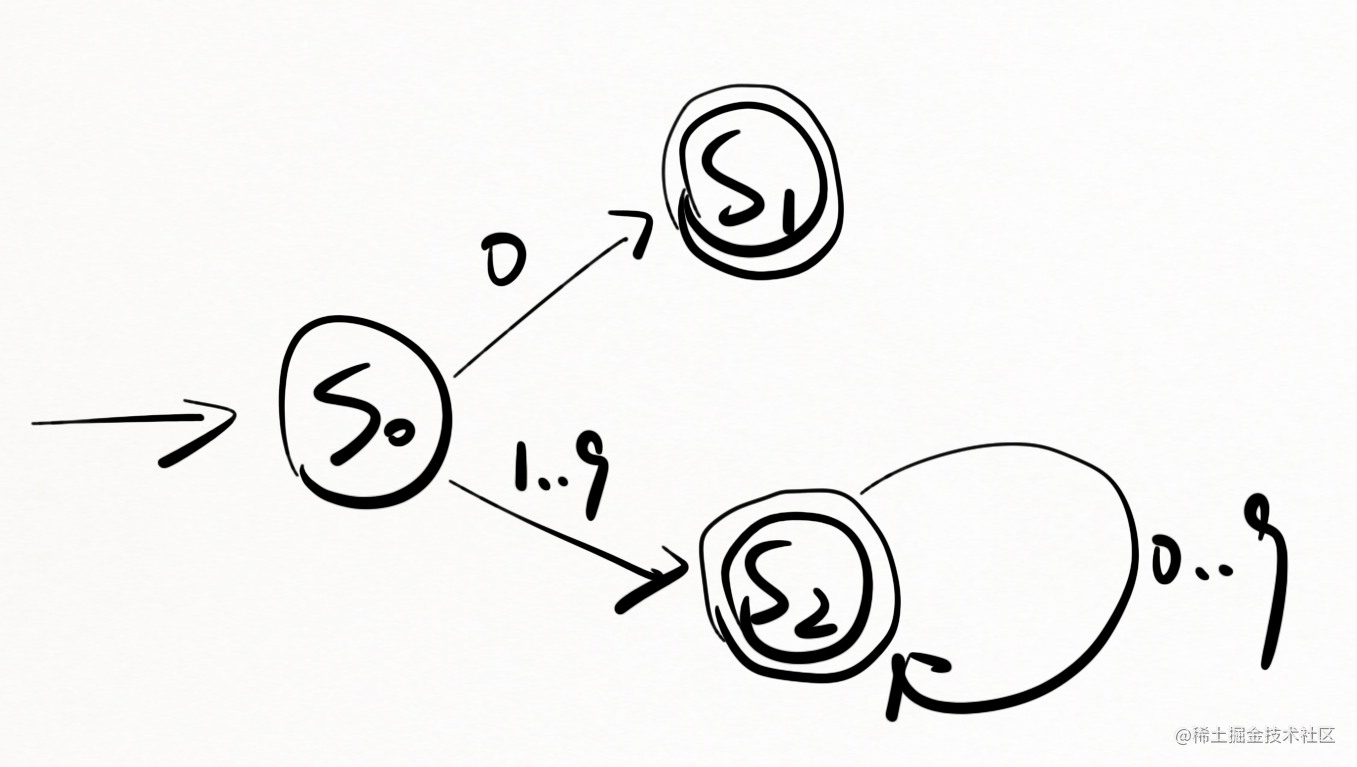## 识别标识符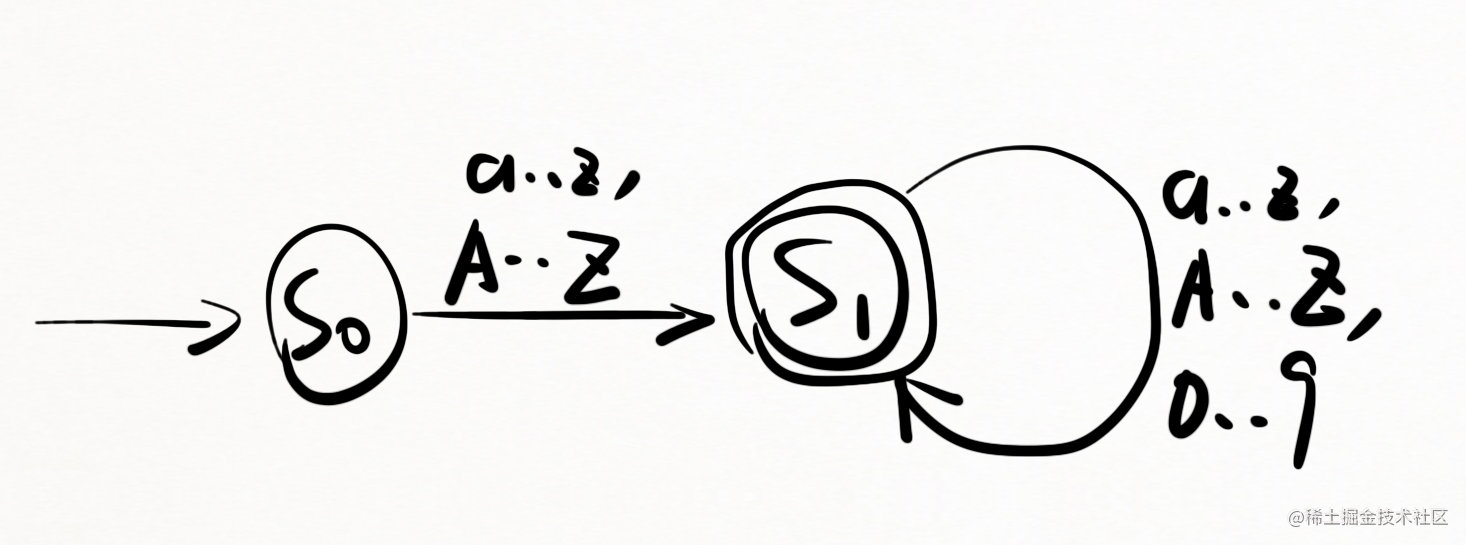# 正则表达式

## 形式化定义

1. 选择，语法为 $R|S$，定义为 ${x | x \in R 或 x \in S }$

2. 连接，语法为 $RS$，定义为 ${xy | x \in R 且 y \in S }$

3. 闭包，语法为 $R*$，定义为 ${ \phi | R | RR | RRR | RRRR | ... }$

• 正闭包，语法为 $R+$，其本质是 $RR*$

[a-zA-Z]([a-zA-Z0-9])*

# 从正则表达式到确定性有限状态机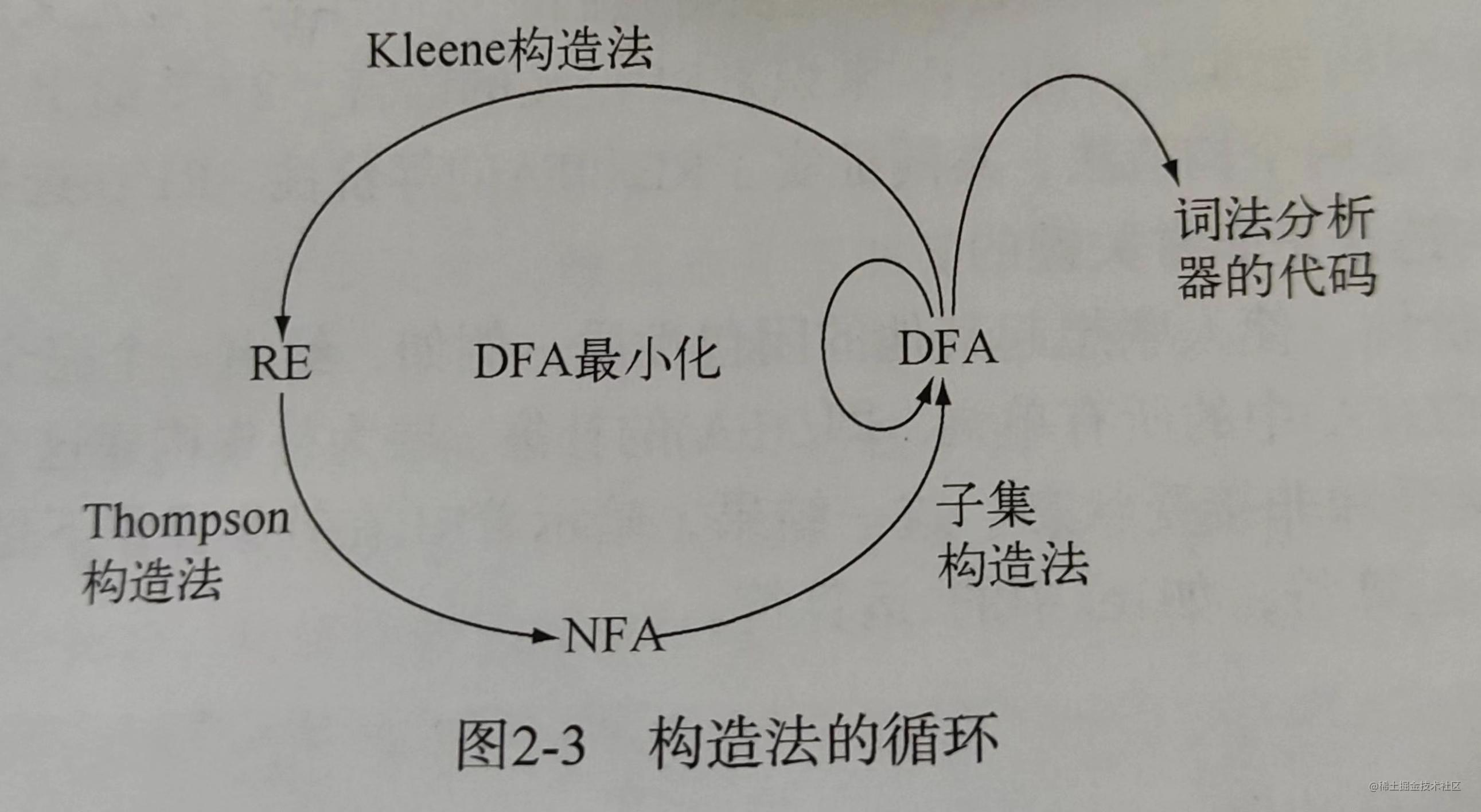1. NFA 允许在 $\epsilon$（空字符串）上进行转移。
2. DFA 不允许。

1. RE => NFA：Thompson 构造法可以把正则表达式变成 NFA
2. NFA => DFA：子集构造法可以把 NFA 变成 DFA
3. DFA => min DFA：Hopcroft 算法或 Brzozowski 算法可以把 DFA 简化成最小 DFA（最小是指状态数最小）
4. 推论：通过上面三个算法，我们可以把正则表达式变成最小 DFA
5. DFA 变成识别器代码是很容易的事情（下节讲）
6. 推论：只要用正则描述一门语言，就可以很容易的得到识别器的代码
7. DFA => RE：Kleene 构造法可以把 DFA 变成正则表达式
8. 推论：DFA 和正则表达式是等价的

# 从 DFA 到代码

1. 表驱动
2. 直接编码
3. 手工编码

## 表驱动的词法分析器

prefixnumberotherother

prefixnumberother
$s_0$$s_1$$s_{error}$$s_{error}$
$s_1$$s_{error}$$s_2$$s_{error}$
$s_2$$s_{error}$$s_2$$s_{error}$
$s_e$$s_{error}$$s_{error}$$s_{error}$

$s_0$$s_1$$s_2$$s_3$

function nextWord(){
let state = 's_0'
let lexeme = ''
let stack = []
while (state !== 's_error') {
nextChar(char) // 此函数以后介绍
lexeme += char
if(['s_2'].include(state)){
stack = []
}
stack.push(state)
categoty = 字符分类表[char]
state = 转移表[state][category]
}
// 如果最终 state 不是接受状态，则回滚到之前的接受状态（如果有）
while (!['s_2'].include(state) && state !== 'bad'){
state = stack.pop()
truncate(lexeme)
rollback() // 此函数以后介绍
}

if(['s_2'].include(state)){
return 结果类型表[state]
} else {
return '无效'
}

}

## 直接编码的词法分析器

while (state !== 's_error') {
nextChar(char) // 此函数以后介绍
...
categoty = 字符分类表[char]
state = 转移表[state][category]
}

goto s_1
}else{
goto s_out
}
s_1:
nextChar(char)
lexeme += char
if(['s_2'].include(state)){
stack = []
}
stack.push(state)
if('0123456789'.include(char)){
goto s_2
}else{
goto s_out
}
s_2:
nextChar(char)
lexeme += char
if(['s_2'].include(state)){
stack = []
}
stack.push(state)
if('0123456789'.include(char)){
goto s_2
}else{
goto s_out
}
s_out:
white(!['s_2'].include(state) && state !== 'bad'){
state = stack.pop()
truncate lexeme
rollback()
}

if(['s_2'].include(state)){
return 结果类型表[state]
} else {
return '无效'
}

1. 违反了结构化编程原则，因为使用了 goto
2. 不适合人类阅读

## 手工编码的词法分析器

buffer 越大，词法分析器读取输入字符串的次数就越少。当然 buffer 也不能无限大。